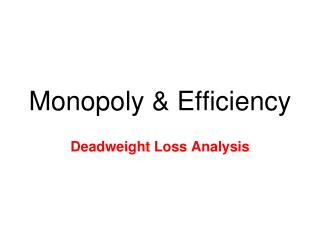# Monopoly & Efficiency - PowerPoint PPT PresentationDownload PresentationMonopoly & Efficiency

Monopoly & EfficiencyDownload Presentation## Monopoly & Efficiency

- - - - - - - - - - - - - - - - - - - - - - - - - - - E N D - - - - - - - - - - - - - - - - - - - - - - - - - - -
##### Presentation Transcript

1. Monopoly & Efficiency Deadweight Loss Analysis

2. Allocative Efficiency (P = MC) • Total Welfare is maximized only when MC = MB for society • Since MB = Price => only when Price = MC • Allocate efficiency is when P = MC • Competitive firms always are allocative efficient (P = MC) • This is considered “socially optimal” (no DWL) • Any other production point produces a deadweight loss • Monopolies are notallocatively efficient (P > MC) • Since P ≠ MC => DWL exists

3. MC Deadweight Loss Allocative Efficiency P = MC Where a competitive industry would produce Monopoly price MR D Monopoly Efficient quantity quantity Inefficiency of Monopoly Price EM EC Quantity 0

4. Deadweight Loss • Deadweight loss is caused by a monopoly, a tax and a subsidy • Unless tax or subsidy is correcting a market failure! • There is never a deadweight loss when P = MC (MB = MC) • If P > MC => market is too small • Example: misplaced tax or monopoly • If P < MC => market is too big • Example: misplaced subsidy

5. Excess profit => some consumer surplus turns into monopoly profit Deadweight Loss as P > MC Deadweight Loss Analysis • Revenue from a tax is transferred from producer/consumer => to Government • Revenue from a subsidy is transferred from Gov’t => to producer/consumer • Monopoly excess profit is transferred from consumer surplus => to private firm Monopoly Price PM ----------------- --------------------- - PC Competitive Price --------------------------- ------------------------ QM QC

6. Monopoly Perfect Competition P > MC P > min of ATC P = MC (always) P = min of ATC (long run) Efficiency Analysis • Allocative Efficiency whenP = MC • Monopolies fail as P > MC • Competitive Firms always are Allocatively Efficient • Production Efficiency whenP = min. of ATC • Monopolies fail as P > min of ATC • Competitive Firms achieve it only in long run

7. Welfare is Maximized! Marginal cost Value MC = MB to buyers Demand Cost (marginal benefit: value to buyers) to monopolist Value to buyers Value to buyers is greater than is less than cost to seller. cost to seller. Efficient Quantity MC = MB Allocative Efficiency Price Quantity 0

8. Worksheet • Monopoly & Deadweight Loss